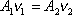The volume flow rate at one position of a pipe equals the volume flow rate at another position in the same pipe.

This is another statement of the continuity equation. It applies to an incompressible fluid flowing through a pipe that is completely full of the fluid. It states that the product of the cross-sectional area and the fluid velocity at one position of the pipe is equal to the product of the same quantities at any other position in the pipe.

Note If you multiply both sides of this equation by the fluid density, one obtains the continuity equation in its more basic form: the mass flow rate at position 1 in the pipe is equal to the mass flow rate at position 2 in the pipe.

Note: The product of the cross-sectional area of the pipe and the veloctiy of the fluid is referred to as the volume flow rate. It also goes by the name of flow rate, or, particularly when rivers are under consideration, discharge. In SI units, it is the number of cubic-meters-per-second of fluid passing a given position on the path of the fluid (m3/s). Units often used in the United States for flow rate are gpm (gallons per minute). No one symbol (we use the product Av) is used to represent volume flow rate on your formula sheet. This practice is fairly standard. Authors that do use a symbol to represent volume flow rate typically use the symbol Q. In such textbooks Q = Av by definition and the formula at the top of this page appears as Q1 = Q2 .

Tip: The form of the continuity equation given here (at the top of this page) is the most useful form for solving the kinds of problems one encounters in an introductory physics course.

Pitfall Avoidance Note: Fluid flow problems often involve the application of this small, simple, but important equation in addition to the application of Bernoulli's equation. Such problems cannot be solved by means of Bernoulli's equation alone.